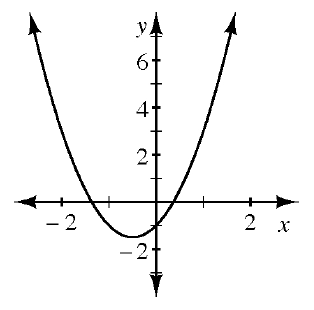### Home > CAAC > Chapter 8 > Lesson 8.2.5 > Problem8-87

8-87.

Examine the graph of $y=2x^2+2x−1$ at right.

1. Estimate the zeros $y=2x^2+2x−1$ from the graph.

Where are the $x$-intercepts of this graph?

2. What happens if you try to use the Zero Product Property to find the roots of $2x^2+2x−1=0$?

What makes the Zero Product Property difficult to use here?